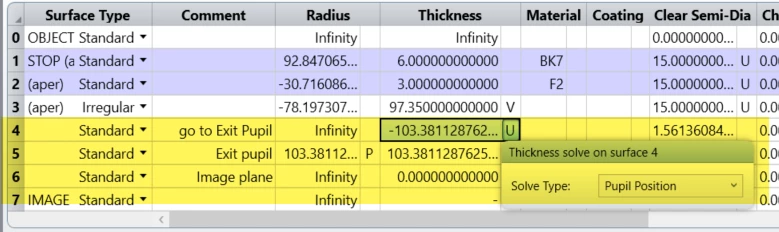Solved

# Display reference sphere on a layout plot

• 4 replies
• 73 views

• Monochrome

Hi all.

I want to display reference sphere on a layout plot.

Is it possible?

Then, could you tell me how can I do this?

icon

Best answer by Sandrine Auriol 7 July 2021, 11:38

View original

### 4 replies

Userlevel 5+2

Sorry it is a bit of a late answer but by reference sphere, do you mean the reference sphere for the wavefront calculation?

If yes you can add two surfaces before the image plane. This will work for on-axis fields. For off-axis, you will need to add some coordinate breaks.Sandrine

Sorry it is a bit of a late answer but by reference sphere, do you mean the reference sphere for the wavefront calculation?

If yes you can add two surfaces before the image plane. This will work for on-axis fields. For off-axis, you will need to add some coordinate breaks.Sandrine

Hello, Sandrine Auriol. Your answer gave me a lot of inspiration. I would like to ask a further question: Does the data of this reference sphere read the optical path of surface 5 in the above figure through the OPTH function? Then OPDC (optical path difference) is the optical path of the exit pupil surface minus the optical path of surface 5 through the OPTH function?
I would be very grateful if you could answer me

Best, Nancy Yang

Userlevel 5+2

Hi Nancy

Yes. In that example, the calculation is performed in the merit function to show how it works. So the OPD plot for the on-axis field is:

Now let’s say I want to recalculate the OPD for the marginal ray so HX=HY=0 and PX=0, PY=1:

So PLEN calculates the optical path length up to surface 4 (Image plane): line 2 is for the chief ray and line 3 is for the marginal ray. So we substract these two values and it gives the optical path difference between the marginal and chief ray up to the image plane.

Then we calculate a correction term. Lines 6 and 7 are the optical paths of the chief and marginal rays from the image plane to the reference sphere (surface 5). Then we compute the difference so it gives the optical path difference between the marginal and chief ray up from the image plane to the reference sphere.

Finally we substract the correction term to the optical path difference between the marginal and chief ray up to the image plane. This is our OPD.

If the system is perfect, all the rays are “like” coming from the reference sphere. When a system is not perfect, the OPD gives us a good idea of the “deformation” from that reference sphere.

I hope it helps but do not hesitate if you have any further questions.

Sandrine

Hi Sandrine

Thank you for your help, this question you have already answered me in the same way under another topic, they are very useful to me, thanks again!

Best，Nancy